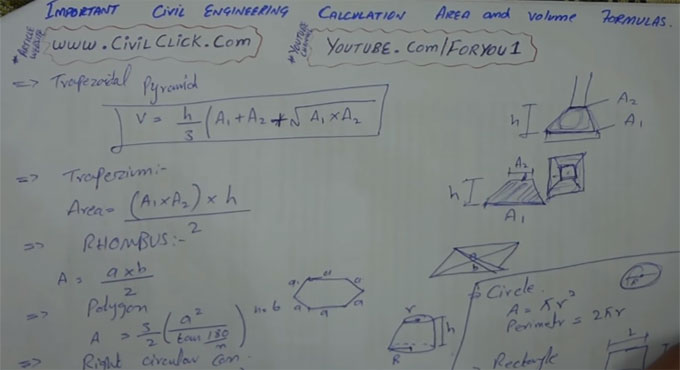# How to calculate volume and area of various complicated geometric shapesIn this construction video tutorial, you will be familiar with various formulas which are essential to measure the area and volume of various complicated geometric shapes.

These complicated shapes range from Trapezoidal pyramid, Right circular cone, Rumbas, Trapezium, Polygon, Circle, Rectangle, Square etc.

Geometry belongs to a section of mathematics that takes care of shape, size, relative position of figures, and the properties of space. It provides a practical means of managing lengths, area and volumes efficiently.

Geometry is segregated into two dissimilar types: Plane Geometry and Solid Geometry. The Plane Geometry is suitable for various shapes like circles, triangles, rectangles, square and more. On the other hand, solid geometry is associated with working out the length, perimeter, area and volume of different geometric figures and shapes. Besides, they are utilized to measure the arc length and radius etc.

Geometry Formula is useful for making calculation of the length, perimeter, area and volume of different geometric shapes and figures. There exist several geometric formulas, which are associated with height, width, length, radius, perimeter, area, surface area or volume and much more.

Given below, an extensive lists of different types of basic geometry formulas :-

Perimeter of a Square=P=4a
Here, a denotes length of the sides of a square

Perimeter of a Rectangle = P = 2(l+b)
Here, l denotes Length and b denotes Breadth

Area of a Square=A=a2
Here, a denotes length of the sides of a square

Area of a Rectangle=A=l×b
Here, l denotes Length and b denotes Breadth

Area of a Triangle=A=b×h2
Here, b denotes base of the triangle and h denotes height of the triangle

Area of a Trapezoid=A=(b1+b2)h2
Where, b1 & b2 belong to the bases of the Trapezoid ; h = height of the Trapezoid

Area of a Circle=A=π×r2
Circumference of a Circle=A=2πr
Here, r denotes Radius of the Circle

Surface Area of a Cube=S=6a2
Here, a denotes length of the sides of a Cube

Surface Area of a Cylinder=S=2πrh
Volume of a Cylinder=V=πr2h
Here, r denotes Radius of the base of the Cylinder and h denotes Height of the Cylinder

To learn the detail calculation process, go through the following construction video tutorial.

Video Source: F&U-FORYOU